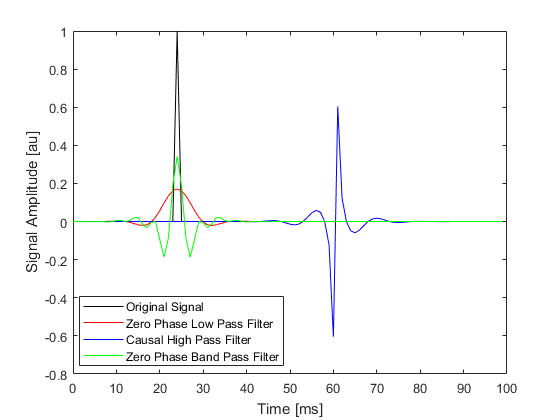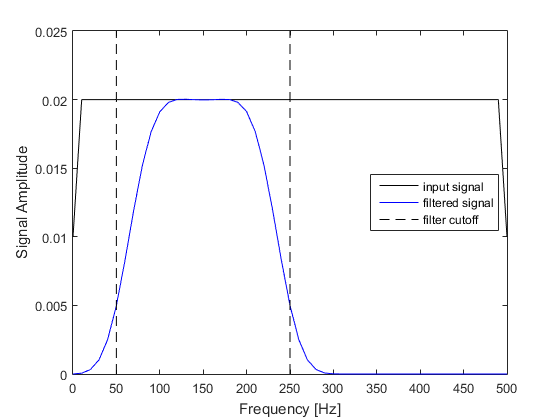# applyFilter

Filter input with high or low pass filter.

## Syntax

```filtered_signal = applyFilter(signal, Fs, cutoff_f, filter_type)
filtered_signal = applyFilter(signal, Fs, cutoff_f, filter_type, ...)
```

## Description

`applyFilter` filters an input signal using `filter`. The FIR filter coefficients are based on a Kaiser window with the specified cut-off frequency and filter type (`'HighPass'`, `'LowPass'` or `'BandPass'`). Both causal and zero phase filters can be applied. An example of using `applyFilter` is given below.

```% create a time series with a single monopolar pulse
N = 100;
dt = 1e-3;
t = 0:dt:dt * (N-1);
pulse = zeros(length(t), 1);
pulse(25) = 1;

% filter using applyFilter
pulse_filtered_lp = applyFilter(pulse, 1/dt, 100, 'LowPass', 'Plot', true, 'ZeroPhase', true);
pulse_filtered_hp = applyFilter(pulse, 1/dt, 100, 'HighPass', 'Plot', true);
pulse_filtered_bp = applyFilter(pulse, 1/dt, [50, 250], 'BandPass', 'Plot', true, 'ZeroPhase', true);

% plot the filtered time series
figure;
[~, scale, prefix] = scaleSI(max(t));
plot(t * scale, pulse, 'k-', ...
t * scale, pulse_filtered_lp, 'r-', ...
t * scale, pulse_filtered_hp, 'b-', ...
t * scale, pulse_filtered_bp, 'g-');
xlabel(['Time [' prefix 's]']);
ylabel('Signal Amplitude [au]');
legend('Original Signal', 'Zero Phase Low Pass Filter', 'Causal High Pass Filter', 'Zero Phase Band Pass Filter', 'Location', 'best');
```## Inputs

 `func` signal to filter `Fs` sampling frequency [Hz] `cutoff_f` filter cutoff frequency/s [Hz] `filter_type` `'HighPass'`, `'LowPass'` or `'BandPass'`

## Optional Inputs

Optional 'string', value pairs that may be used to modify the default computational settings.

Input Valid Settings Default Description
`'Plot'` (Boolean scalar) `false` Boolean controlling whether the amplitude spectrum is displayed before and after filtering.
`'StopBandAtten'` (numeric scalar) `60` Attenuation in decibels in the stop band.
`'TransitionWidth'` (numeric scalar) `0.1` Size of the transition based on the temporal sampling frequency.
`'ZeroPhase'` (Boolean scalar) `false` Boolean controlling whether a zero phase filter is used.

## Outputs

 `filtered_signal` the filtered signal

## Examples

`filterTimeSeries`